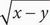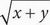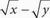# SAT Math Multiple Choice Question 1: Answer and Explanation

### Test Information

Question: 1

1. Which of the following represents the statement “the sum of the squares of x and y is equal to the square root of the difference of x and y”?

• A. x2 + y2 =• B. x2y2 =• C. (x + y)2 =• D.= (xy)2